Home > INT3 > Chapter 11 > Lesson 11.2.4 > Problem11-112

11-112.
1. Solve the following equations. Write your answers in exact form and approximate form, accurate to three decimal places. Homework Help ✎

1. –4x5 – 6 = –1

2. –7(10x) + 9 = –5

3. 8log7(1 + x) – log7(1) = 7

4.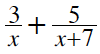= –2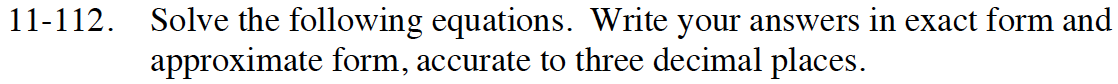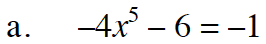$x=\sqrt{\frac{-5}{4}}$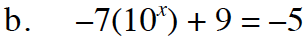10x = 2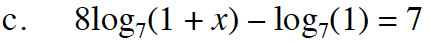log7(1) = 0

8 log7(1 + x) = 7 ⇒ log7 (1 + x)8 = 7

x = 77/8 − 1 ≈ 4.489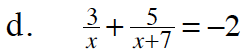Use fraction busters to clear the fractions and then solve the quadratic equation.